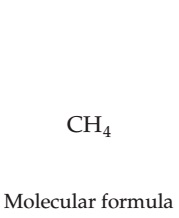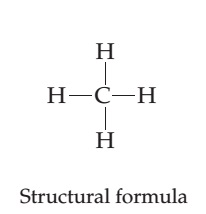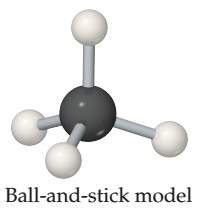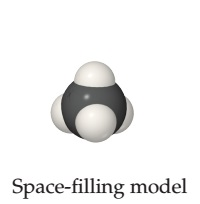# Problem: Different representations of the methane (CH4) molecule. Structural formulas, perspective drawings, ball-and-stick models, and space-filling models.Which model, the ball-and-stick or the space-filling, more effectively shows the angles between bonds around a central atom?

###### FREE Expert Solution

Analyze each representation of CH4 and determine which effectively represents the 3D or ball-and-stick model

Recall that molecules are 3D objects which means that we can't properly model it using a paper (2D)

95% (177 ratings)###### Problem Details

Different representations of the methane (CH4) molecule. Structural formulas, perspective drawings, ball-and-stick models, and space-filling models.Which model, the ball-and-stick or the space-filling, more effectively shows the angles between bonds around a central atom?